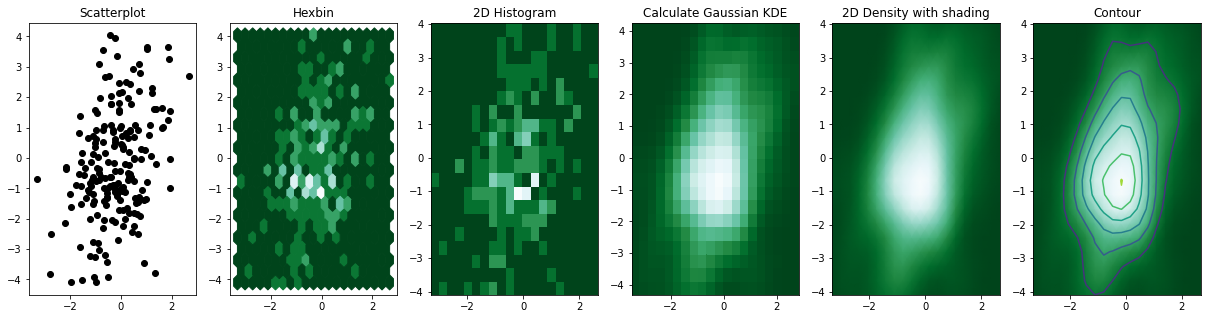Consider the scatterplot on the left hand side of this figure. A lot of dots overlap and they make the figure hard to read. Even worse, it is impossible to determine how many data points are in each position. In this case, a possible solution is to cut the plotting window into several bins, and represent the number of data points in each bin by a color. Following the shape of the bin, this makes Hexbin plot or 2D histogram.

Then, it is possible to make a smoother result using Gaussian KDE (kernel density estimate). Its representation is called a 2D density plot, and you can add a contour to denote each step. You can see more examples of these types of graphics in the 2D density section of the python graph gallery. This plot has been inspired by this stack overflow question.

``````# Libraries
import numpy as np
import matplotlib.pyplot as plt
from scipy.stats import kde

# Create data: 200 points
data = np.random.multivariate_normal([0, 0], [[1, 0.5], [0.5, 3]], 200)
x, y = data.T

# Create a figure with 6 plot areas
fig, axes = plt.subplots(ncols=6, nrows=1, figsize=(21, 5))

# Everything starts with a Scatterplot
axes.set_title('Scatterplot')
axes.plot(x, y, 'ko')
# As you can see there is a lot of overlapping here!

# Thus we can cut the plotting window in several hexbins
nbins = 20
axes.set_title('Hexbin')
axes.hexbin(x, y, gridsize=nbins, cmap=plt.cm.BuGn_r)

# 2D Histogram
axes.set_title('2D Histogram')
axes.hist2d(x, y, bins=nbins, cmap=plt.cm.BuGn_r)

# Evaluate a gaussian kde on a regular grid of nbins x nbins over data extents
k = kde.gaussian_kde(data.T)
xi, yi = np.mgrid[x.min():x.max():nbins*1j, y.min():y.max():nbins*1j]
zi = k(np.vstack([xi.flatten(), yi.flatten()]))

# plot a density
axes.set_title('Calculate Gaussian KDE')

# contour
axes.set_title('Contour')
``<matplotlib.contour.QuadContourSet at 0x7fa6d5404390>``